## CROSSHATCH

 Applies a crosshatch effect to an image.

 USAGE: crosshatch [-l length] [-s sharp ] [-g gain ] [-a amount] [-p prune] [-b balance] [-e effect] [-m mix] [-B bri] [-C con] [-S sat] infile outfile USAGE: crosshatch [-h|-help] -l ... length .... length of strokes; integer>=0; default=7 -s ... sharp ..... sharpness; integer>=0; default=10 -g ... gain .......gain (strength) of strokes; integer>=0; default=1 -a ... amount .... edge amount; integer>0; default=1 -p ... prune ..... edge pruning; integer (positive or negative); default=0 -b ... balance ... balance between plus and minus 45 degree diagonal strokes; .................. -100<=integer<=100; default=0 -e ... effect .... stroke effect; choices are normal(n), light(l) or dark(d); .................. default=normal -m ... mix ....... mixing (blend) between original and processing image; .................. 0<=integer<=100; 0 is original image, 100 is processed .................. image; default=100 (processed image) -B ... bri ....... percent change of brightness; positive or negative integer; .................. default=0 -C ... con ....... percent change of contrast; positive or negative integer; .................. default=0 -S ... sat ....... percent change of saturation; positive or negative integer; .................. default=0 PURPOSE: To apply a crosshatch effect to an image. DESCRIPTION: CROSSHATCH applies a crosshatch effect to an image. Options include: the length, gain, (edge) amount, balance and effect of the stroke. ARGUMENTS: -l length ... LENGTH of strokes. Values are integers>=0. The default=7. -s sharp ... SHARP is the sharpness of image and strokes. Values are integers>=0. The default=10. -g gain ... GAIN (strength) of strokes. Values are integers>=0. The default=1. -a amount ... EDGE AMOUNT. Values are integers>0. The default=1. -p prune ... EDGE PRUNING. Values are integers (positive or negative). Positives remove lighter edges and negatives remove darker edges. The default=0. -b balance ... BALANCE between plus and minus 45 degree diagonal strokes. Values are -100<=integer<=100. The default=0. -e effect ... STROKE EFFECT. The choices are normal(n), light(l) or dark(d). The default=normal. -m mix ... MIX (blend) between original and processing image. Values are 0<=integer<=100. 0 is original image. 100 is processed image. The default=100 (processed image). -B bri... BRI is the percent change of brightness. Values are positive or negative integers. The default=0. -C con... CON is the percent change of contrast. Values are positive or negative integers. The default=0. -S sat... SAT is the percent change of saturation. Values are positive or negative integers. The default=0. CAVEAT: No guarantee that this script will work on all platforms, nor that trapping of inconsistent parameters is complete and foolproof. Use At Your Own Risk.

### EXAMPLES

 Example 1 - Variation In Sharpening OriginalArguments: -l 7 -s 0 -g 0 -a 1 Arguments: -l 7 -s 5 -g 0 -a 1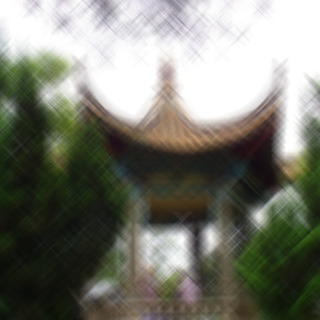Arguments: -l 7 -s 10 -g 0 -a 1 Arguments: -l 7 -s 20 -g 0 -a 1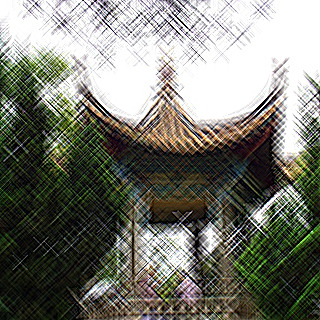Example 2 - Variation In Gain OriginalArguments: -l 7 -s 10 -g 1 -a 1 Arguments: -l 7 -s 10 -g 3 -a 1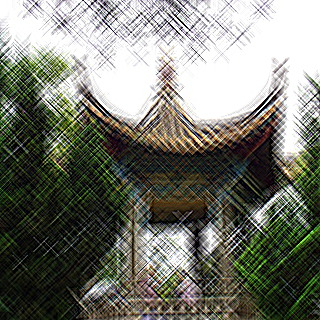Arguments: -l 7 -s 10 -g 5 -a 1 Arguments: -l 7 -s 10 -g 10 -a 1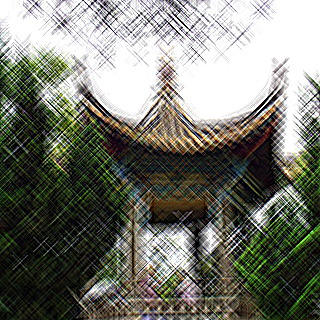Example 3 - Variation In Amount; Gain = 1 OriginalArguments: -l 7 -s 10 -g 1 -a 1 Arguments: -l 7 -s 10 -g 1 -a 2Arguments: -l 7 -s 10 -g 1 -a 5 Arguments: -l 7 -s 10 -g 1 -a 10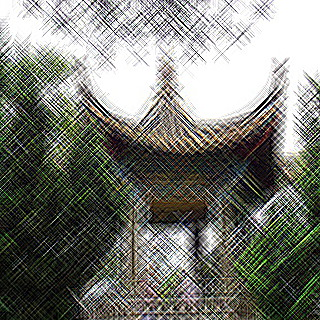Example 4 - Variation In Amount; Gain = 5 OriginalArguments: -l 7 -s 10 -g 5 -a 1 Arguments: -l 7 -s 10 -g 5 -a 3Arguments: -l 7 -s 10 -g 5 -a 5 Arguments: -l 7 -s 10 -g 5 -a 10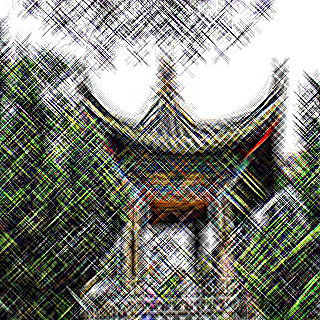Example 5 - Variation In Pruning OriginalArguments: -l 7 -s 10 -g 1 -a 1 -p -2 Arguments: -l 7 -s 10 -g 1 -a 1 -p 0 Animation -l 7 -s 10 -g 1 -a 1 -p 2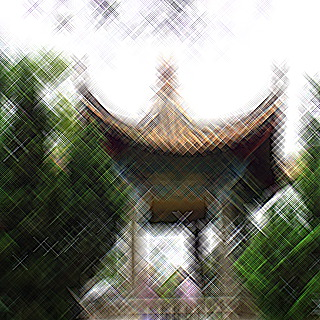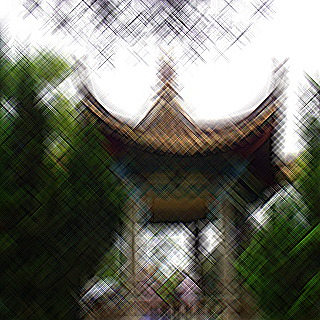Example 6 - Variation In Balance OriginalArguments: -l 7 -s 10 -g 1 -a 1 -b -100 Arguments: -l 7 -s 10 -g 1 -a 1 -b 0 Animation -l 7 -s 10 -g 1 -a 1 -b 100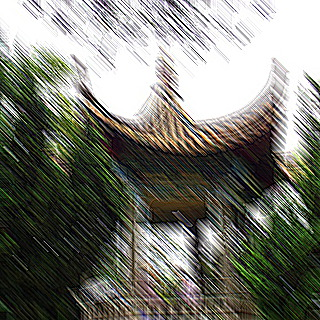Example 7 - Variation In Effect OriginalArguments: -l 7 -s 10 -g 1 -a 1 -e dark Arguments: -l 7 -s 10 -g 1 -a 1 -e normal Animation -l 7 -s 10 -g 1 -a 1 -e light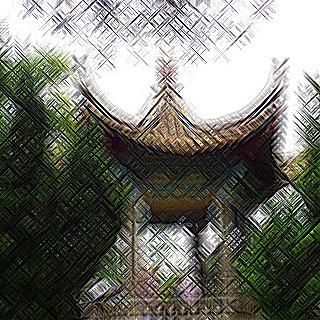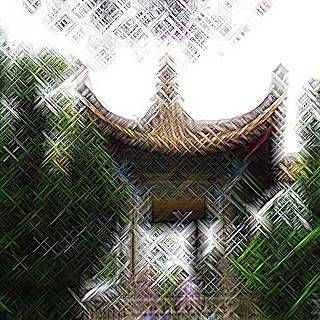What the script does is as follows: Applies unsharp masking to sharpen the image using gain and amount arguments Applies a linear diagonal blur convolution on the sharpened image at each of plus and minus 45 degrees and the desired length Blends the two previous blurred results at the desired balance Applies unsharp masking to that result using the sharp argument Blends the original with the processed image Applies any desired brightness, contrast or saturation This is equivalent to the following IM commands length=\$((2*length+1)) convert \$infile -unsharp 0x\${gain}+\${amount}+0 \$dir/tmpS.mpc apply diagBlurPlus function to do linear convolution along one diagonal apply diagBlurMinus function to do linear convolution along the other diagonal balance1=`convert xc: -format "%[fx:(\$balance+100)/2]" info:` balance2=\$((100-\$balance1)) convert \$dir/tmpB45.mpc \$dir/tmpBm45.mpc -compose blend \ -define compose:args=\$balance2,\$balance1 -composite \$dir/tmpB.mpc convert \$dir/tmpB.mpc -unsharp 0x1+\${sharp}+0 \$dir/tmpBs.mpc convert \$infile \$dir/tmpBs.mpc \ -compose blend -define compose:args=\$mix -composite \ -brightness-contrast \$bri,\$con -modulate 100,\$sat,100 \ \$outfile